# Colligative Properties of SolutionsPage 1

#### WATCH ALL SLIDES

Slide 1Colligative Properties of Solutions

Jacobus Henricus van 't Hoff

(1852-1911)

Slide 2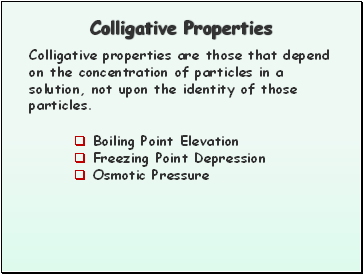## Colligative Properties

Colligative properties are those that depend on the concentration of particles in a solution, not upon the identity of those particles.

Boiling Point Elevation

Freezing Point Depression

Osmotic Pressure

Slide 3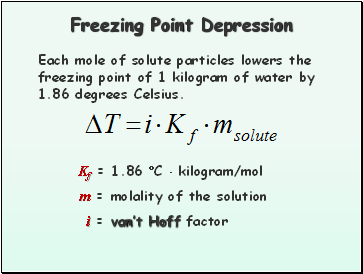## Freezing Point Depression

Each mole of solute particles lowers the freezing point of 1 kilogram of water by 1.86 degrees Celsius.

Kf = 1.86 C  kilogram/mol

m = molality of the solution

i = van’t Hoff factor

Slide 4## Boiling Point Elevation

Each mole of solute particles raises the boiling point of 1 kilogram of water by 0.51 degrees Celsius.

Kb = 0.51 C  kilogram/mol

m = molality of the solution

i = van’t Hoff factor

Slide 5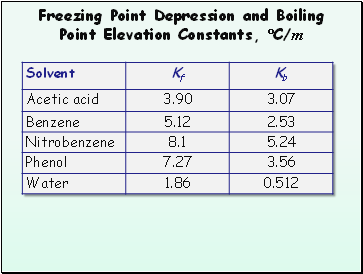Freezing Point Depression and Boiling Point Elevation Constants, C/m

Slide 6## The van’t Hoff Factor, i

Electrolytes may have two, three or more times the effect on boiling point, freezing point, and osmotic pressure, depending on its dissociation.

Slide 7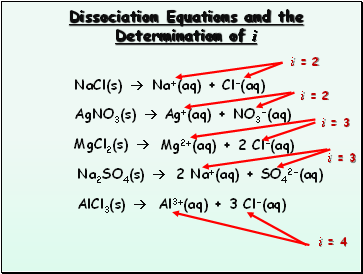## Dissociation Equations and the Determination of i

NaCl(s) 

AgNO3(s) 

MgCl2(s) 

Na2SO4(s) 

AlCl3(s) 

Na+(aq) + Cl-(aq)

Ag+(aq) + NO3-(aq)

Mg2+(aq) + 2 Cl-(aq)

2 Na+(aq) + SO42-(aq)

Al3+(aq) + 3 Cl-(aq)

i = 2

i = 2

i = 3

i = 3

i = 4

Slide 8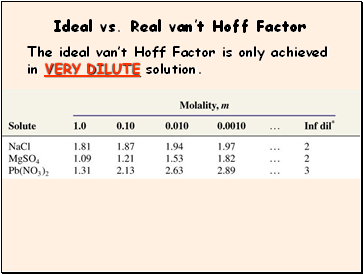## Ideal vs. Real van’t Hoff Factor

The ideal van’t Hoff Factor is only achieved in VERY DILUTE solution.

Slide 9## Osmotic Pressure

The minimum pressure that stops the osmosis is equal to the osmotic pressure of the solution

Slide 10Osmotic Pressure Calculations

 = Osmotic pressure

M = Molarity of the solution

R = Gas Constant = 0.08206 Latm/molK

i = van’t Hoff Factor